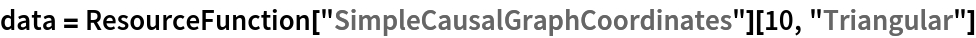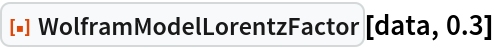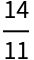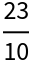Function Repository Resource:

# WolframModelLorentzFactor

Calculate the Lorentz factor of a Lorentz-transformed Wolfram Model causal graph

Contributed by: Tom Lee, Jon Lederman
 ResourceFunction["WolframModelLorentzFactor"][data,beta] returns the ratio of step sizes of a beta-transformed causal graph and the initial graph given by data.

## Details

data is a causal data coordinate data set. It consists of triples of the form {index,time,space}, where the second and third coordinates denote initial values in Minkowski 1+1 dimensional space-time. This can be generated using ResourceFunction["SimpleCausalGraphCoordinates"].
The graph is Lorentz transformed using beta as the ratio of velocity to the light speed, that is, v/c.

## Examples

### Basic Examples (2)

Construct a simple triangular shape causal graph with initial node size 10:

 In:=Out=Calculate the Lorentz factor at v/c=0.3:

 In:=Out=### Scope (1)

Calculate the Lorentz factor for a causal graph on a hexagonal grid, at v/c=0.8:

 In:=Out=Tom Lee

## Version History

• 1.0.0 – 17 June 2022